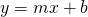# Linear Relations: Rate of Change, Initial Value

Appliance Repair

Here is a set of points showing the relationship between how long an appliance repair technician works in a home and how much their visit costs.

(a) How much does it cost to have the technician arrive at your house?

(b) How much does the fee increase for each hour?

A rate of change is the increase of the dependent variable (vertical increment) for every one unit of increase of the independent (horizontal increment) variable.

(c) In this context, we describe the rate of change as: the cost of the technician’s time increases by …. dollars for every one hour spent working.

(d) If you were to write this relationship in the formwhat is the second element of the ordered pair in terms of?

(e) What equation can you write in the formthat goes through these points?

Try your equation out in the applet above.

Truck Rental

Here is a set of points showing the relationship between the how far a cargo truck is driven and how much it costs to rent it.

(a) How much does it cost to rent the truck and to not drive it anywhere?

(b) How much does the price increase every 10 km driven?

(c) How much does the price increase for every 1 km driven?

A rate of change is the increase of the dependent variable (vertical increment) for every one unit of increase of the independent (horizontal increment) variable.

(d) In this context we describe the rate of change as: the cost of renting increases by ….. dollars for every one km driven.

(e) If you were to write this relationship in the formwhat is the second element of the ordered pair be in terms of?

(f) What equation can you write in the formthat goes through these points?

Try your equation out in the applet above.